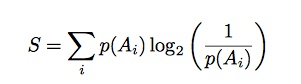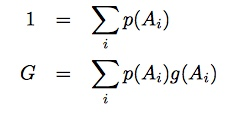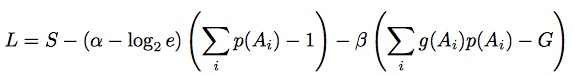# Maximum Entropy Principle: Definition

Share on

The maximum entropy principle is a rule which allows us to choose a ‘best’ from a number of different probability distributions that all express the current state of knowledge. It tells us that the best choice is the one with maximum entropy.

This will be the system with the largest remaining uncertainty, and by choosing it you’re making sure you’re not adding any extra biases or uncalled for assumptions into your analysis.

We know that all systems tend toward maximal entropy configurations over time, so the likelihood that your system is accurately represented by the maximum entropy distribution is higher than the likelihood it would be represented by a more ordered system.

## Applying the Maximum Entropy Principle

Applying the maximum entropy principle to a physical problem typically involves algebraically solving a series of equations for a number of unknowns.

For instance, consider the discrete case where you’d like to find out the probability of a quantity taking on values {a, b, c, d…}. The probabilities will all add up to one; so your first equation is p(a) + p(b) + p(c) + p(d)… = 1.

You may know some information about the situation; if you do, that goes in another equation, called constraints.

## Specific Example

Let’s say your research was on the probability of people buying apples, bananas, or oranges. If they bought one of three in a particular supermarket, you’d know:

1 = Papple + Pbanana + Poranges.

If you know also that apples cost a dollar each, bananas two dollars, and oranges three dollars, and if you know that the average price of fruit bought in the supermarket is \$1.75, you’d know

\$1.75 = \$1.00 Papple + \$2.00 Pbanana + \$3.00 Poranges.

That is what we call our constraint equation. This might be all the information you have. But with two equations and three unknowns, it simply isn’t enough information to come up with a unique solution. That’s where the maximum entropy principle comes in. The maximum entropy principle narrows down the space of all the potentially possible solutions—and there are lots—to the one best solution; the one with the highest entropy.

Call the entropy of the system S. We know that Shannon entropy is defined as:The logarithm, logb(1/p(A)i) represents the information in state i, so when that is multiplied by p(A)i for each i, we get a measure of uncertainty. So our third equation is this one:

S = Papple log2 (1/ Papple) + Pbanana log2 (1/ Pbanana) + Porange log2 (1/ Porange).

Now the rest is just algebra.

### Algebra Steps

Multiplying every term in your first equation by -\$1.00 means that, when you add it to your constraint equation, Papple falls out and you have an equation with just Pbanana and Porange.

\$0.75 = \$1.00Pbanana + \$2.00Porange

so we can write Pbanana in terms of Porange like this:

Pbanana = 0.75 – 2.00 Porange.

We could write Papple in terms of Porange if, instead of multiplying equation 1 by -\$1.00 to begin with, we multiply by -\$2.00. Then we’d we have

-\$0.25 = -\$1.00Papple+ 1.00Porange

which gives us

Papple = Porange + 0.25.

### Final Steps

Now we can go back to our third equation, the one where all the probabilities add up to entropy, and we can write all the other probabilities in terms of Porange using the two equations we just derived

S = (Porange + 0.25) log2 (1/ (Porange0.25)) + (0.75 – 2 Porange )log2 (1/ (0.75- 2Porange)) + Porange log2 (1/ Porange)

Now all that remains is to find the value of Porange so that S is maximized. You can use any of a number of methods to do this; finding the critical points of the function is one good one. We find that entropy is maximized when Porange = (3.25 – √3.8125) /6, which is about 0.216.

Using the equations above, we can conclude that Papple is 0.466, and Pbanana is 0.318.

## Extending the Maximum Entropy Principle to Larger Systems

Adding a consideration of entropy can fully define the situation if we’ve got three variables and only one constraint, as above. But what happens in more complicated situations; for instance, where you have more than three variables? It is a poorly stocked supermarket that only carries oranges, bananas, and apples. What if grapes and cantaloupe were among other options?

It turns out the maximum entropy principle can fully define this situation as well. Besides using the equations used above, you can also use:First, we need to define two new unknowns, α and β these are called the Legrange multipliers. Then we use the Lagrange functionHere, e is the base of the natural log, 2.7183, so log2e is just 1.4427.
To find unknown probabilities in a case like the above, we solve our collection of equations so that L is maximized. This also maximizes our old friend S, entropy.

## References

Penfield, Paul. Information and Entropy Lecture Notes: Chapter 9: Principle of Maximum Energy: Simple Form and Chapter 10: Principle of Maximum Entropy. Retrieved from: https://mtlsites.mit.edu/Courses/6.050/2003/notes/chapter9.pdf chapter10.pdf on February 19, 2018

Xie, Yao. ECE587 Information Theory Lecture Notes: Lecture 11, Maximum Entropy. Retrieved March 1, 2018 from:
https://www2.isye.gatech.edu/~yxie77/ece587/Lecture11.pdf on February 19, 2018

CITE THIS AS:
Stephanie Glen. "Maximum Entropy Principle: Definition" From StatisticsHowTo.com: Elementary Statistics for the rest of us! https://www.statisticshowto.com/maximum-entropy-principle/
---------------------------------------------------------------------------Need help with a homework or test question? With Chegg Study, you can get step-by-step solutions to your questions from an expert in the field. Your first 30 minutes with a Chegg tutor is free!